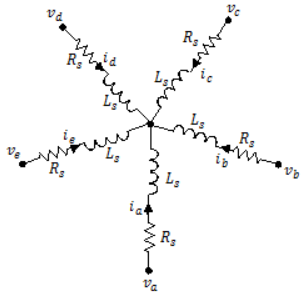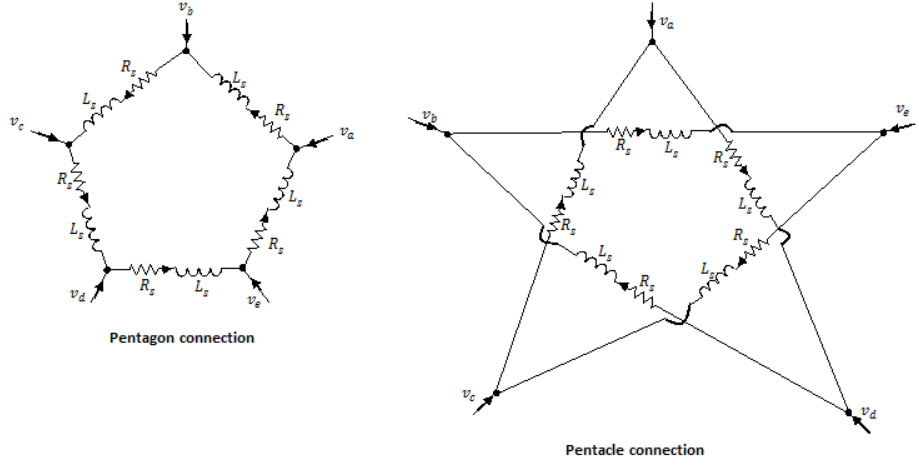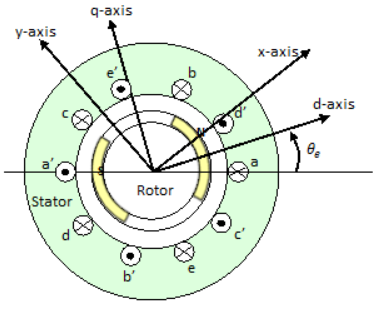# PMSM (Five-Phase)

Five-phase permanent magnet synchronous motor with sinusoidal flux distribution

Since R2020a

•Libraries:
Simscape / Electrical / Electromechanical / Permanent Magnet

## Description

The PMSM (Five-Phase) block models a permanent magnet synchronous motor with a five-phase star-wound stator. The figure shows the equivalent electrical circuit for the star-connected stator windings.You can also model the permanent magnet synchronous motor either in a pentagon-wound or a pentacle-wound configuration by setting Winding type to `Pentagon-wound` or `Pentacle-wound`.### Motor Construction

This figure shows the motor construction with a single pole-pair on the rotor.Permanent magnets generate a rotor magnetic field that creates a sinusoidal rate of change of flux based on the rotor angle.

For the axes convention, when you set the Rotor angle definition parameter to ```Angle between the a-phase magnetic axis and the d-axis```, the a-phase and permanent magnet fluxes align when the rotor mechanical angle, θr, is zero. When you set the Rotor angle definition parameter to ```Angle between the a-phase magnetic axis and the q-axis```, the rotor mechanical angle is the angle between the a-phase magnetic axis and the rotor q-axis.

### Equations

The voltages across the stator windings are

`$\left[\begin{array}{l}{v}_{a}\\ {v}_{b}\\ {v}_{c}\\ {v}_{d}\\ {v}_{e}\end{array}\right]=\left[\begin{array}{ccccc}{R}_{s}& 0& 0& 0& 0\\ 0& {R}_{s}& 0& 0& 0\\ 0& 0& {R}_{s}& 0& 0\\ 0& 0& 0& {R}_{s}& 0\\ 0& 0& 0& 0& {R}_{s}\end{array}\right]\left[\begin{array}{l}{i}_{a}\\ {i}_{b}\\ {i}_{c}\\ {i}_{d}\\ {i}_{e}\end{array}\right]+\left[\begin{array}{l}\frac{d{\psi }_{a}}{dt}\\ \frac{d{\psi }_{b}}{dt}\\ \frac{d{\psi }_{c}}{dt}\\ \frac{d{\psi }_{d}}{dt}\\ \frac{d{\psi }_{e}}{dt}\end{array}\right],$`

where:

• va, vb, vc, vd, and ve are the individual phase voltages across the stator windings.

• Rs is the equivalent resistance of each stator winding.

• ia, ib, ic, id, and ie are the currents flowing in the stator windings.

• $\frac{d{\psi }_{a}}{dt},$$\frac{d{\psi }_{b}}{dt},$$\frac{d{\psi }_{c}}{dt},$ $\frac{d{\psi }_{d}}{dt},$ and $\frac{d{\psi }_{e}}{dt}$ are the rates of change for the magnetic flux in each stator winding.

The permanent magnet and the five windings contribute to the total flux linking each winding. The total flux is

`$\left[\begin{array}{l}{\psi }_{a}\\ {\psi }_{b}\\ {\psi }_{c}\\ {\psi }_{d}\\ {\psi }_{e}\end{array}\right]=\left[\begin{array}{ccccc}{L}_{a}{}_{a}& {L}_{a}{}_{b}& {L}_{a}{}_{c}& {L}_{a}{}_{d}& {L}_{a}{}_{e}\\ {L}_{ba}& {L}_{bb}& {L}_{b}{}_{c}& {L}_{b}{}_{d}& {L}_{b}{}_{e}\\ {L}_{c}{}_{a}& {L}_{c}{}_{b}& {L}_{c}{}_{c}& {L}_{c}{}_{d}& {L}_{c}{}_{e}\\ {L}_{d}{}_{a}& {L}_{d}{}_{b}& {L}_{d}{}_{c}& {L}_{d}{}_{d}& {L}_{d}{}_{e}\\ {L}_{e}{}_{a}& {L}_{e}{}_{b}& {L}_{e}{}_{c}& {L}_{e}{}_{d}& {L}_{e}{}_{e}\end{array}\right]\left[\begin{array}{l}{i}_{a}\\ {i}_{b}\\ {i}_{c}\\ {i}_{d}\\ {i}_{e}\end{array}\right]+\left[\begin{array}{l}{\psi }_{am}\\ {\psi }_{bm}\\ {\psi }_{cm}\\ {\psi }_{dm}\\ {\psi }_{em}\end{array}\right],$`

where:

• ψa, ψb, ψc, ψd, and ψe are the total fluxes that link each stator winding.

• Laa, Lbb, Lcc, Ldd, and Lee are the self-inductances of the stator windings.

• Lab, Lac, Lba, and so on, are the mutual inductances of the stator windings.

• ψam, ψbm, ψcm, ψdm, and ψem are the permanent magnet fluxes linking the stator windings.

The inductances in the stator windings are functions of the rotor electrical angle and defined by

`${\theta }_{e}=N{\theta }_{r}+rotor\text{\hspace{0.17em}}offset$`

`${L}_{aa}={L}_{s}+{L}_{m}\text{cos}\left(2{\theta }_{e}\right)$`

`${L}_{bb}={L}_{s}+{L}_{m}\text{cos}\left(2\left({\theta }_{e}-2\pi /5\right)\right)$`

`${L}_{cc}={L}_{s}+{L}_{m}\text{cos}\left(2\left({\theta }_{e}-4\pi /5\right)\right)$`

`${L}_{dd}={L}_{s}+{L}_{m}\text{cos}\left(2\left({\theta }_{e}+4\pi /5\right)\right)$`

`${L}_{ee}={L}_{s}+{L}_{m}\text{cos}\left(2\left({\theta }_{e}+2\pi /5\right)\right)$`

`${L}_{ab}={L}_{ba}={L}_{ce}={L}_{ec}=-{M}_{s}+{L}_{m}\text{cos}\left(2\left({\theta }_{e}-2\pi /5\right)\right)$`

`${L}_{bc}={L}_{cb}=-{M}_{s}+{L}_{m}\text{cos}\left(2\left({\theta }_{e}-6\pi /5\right)\right)$`

`${L}_{cd}={L}_{dc}={L}_{be}={L}_{eb}=-{M}_{s}+{L}_{m}\text{cos}\left(2{\theta }_{e}\right)$`

`${L}_{de}={L}_{ed}=-{M}_{s}+{L}_{m}\text{cos}\left(2\left({\theta }_{e}+6\pi /5\right)\right)$`

`${L}_{ea}={L}_{ae}={L}_{bd}={L}_{db}=-{M}_{s}+{L}_{m}\text{cos}\left(2\left({\theta }_{e}+2\pi /5\right)\right)$`

`${L}_{ac}={L}_{ca}=-{M}_{s}+{L}_{m}\text{cos}\left(2\left({\theta }_{e}-4\pi /5\right)\right)$`

`${L}_{ad}={L}_{da}=-{M}_{s}+{L}_{m}\text{cos}\left(2\left({\theta }_{e}+4\pi /5\right)\right)$`

where:

• θr is the rotor mechanical angle.

• θe is the rotor electrical angle.

• rotor offset is `pi/2` if you define the rotor electrical angle with respect to the d-axis, or `0` if you define the rotor electrical angle with respect to the q-axis.

• Ls is the stator per-phase self-inductance. This value is the average self-inductance of each of the stator windings.

• Lm is the stator inductance fluctuation. This value is the amount the self-inductance and mutual inductance fluctuate with the changing of the rotor angle.

• Ms is the stator mutual inductance. This value is the average mutual inductance between the stator windings.

The permanent magnet flux linking winding a-a' is at maximum when θe = 0° and zero when θe = 90°. Therefore, the linked motor flux is defined by:

`$\left[\begin{array}{l}{\psi }_{am}\\ {\psi }_{bm}\\ {\psi }_{cm}\\ {\psi }_{dm}\\ {\psi }_{em}\end{array}\right]=\left[\begin{array}{l}{\psi }_{m}\mathrm{cos}{\theta }_{e}\\ {\psi }_{m}\mathrm{cos}\left({\theta }_{r}-2\pi /5\right)\\ {\psi }_{m}\mathrm{cos}\left({\theta }_{r}-4\pi /5\right)\\ {\psi }_{m}\mathrm{cos}\left({\theta }_{r}+4\pi /5\right)\\ {\psi }_{m}\mathrm{cos}\left({\theta }_{r}+2\pi /5\right)\end{array}\right],$`

where ψm is the permanent magnet flux linkage.

### Simplified Electrical Equations

To remove the rotor angle dependence for the inductive terms, you perform a transformation, T, on the motor equations.

The T transformation is defined by:

`$T\left({\theta }_{e}\right)=\frac{2}{5}\left[\begin{array}{ccccc}\mathrm{sin}{\theta }_{e}& \mathrm{sin}\left({\theta }_{e}-2\pi /5\right)& \mathrm{sin}\left({\theta }_{e}-4\pi /5\right)& \mathrm{sin}\left({\theta }_{e}+4\pi /5\right)& \mathrm{sin}\left({\theta }_{e}+2\pi /5\right)\\ \mathrm{cos}{\theta }_{e}& \mathrm{cos}\left({\theta }_{e}-2\pi /5\right)& \mathrm{cos}\left({\theta }_{e}-4\pi /5\right)& \mathrm{cos}\left({\theta }_{e}+4\pi /5\right)& \mathrm{cos}\left({\theta }_{e}+2\pi /5\right)\\ \mathrm{sin}{\theta }_{e}& \mathrm{sin}\left({\theta }_{e}+4\pi /5\right)& \mathrm{sin}\left({\theta }_{e}-2\pi /5\right)& \mathrm{sin}\left({\theta }_{e}+2\pi /5\right)& \mathrm{sin}\left({\theta }_{e}-4\pi /5\right)\\ \mathrm{cos}{\theta }_{e}& \mathrm{cos}\left({\theta }_{e}+4\pi /5\right)& \mathrm{cos}\left({\theta }_{e}-2\pi /5\right)& \mathrm{cos}\left({\theta }_{e}+2\pi /5\right)& \mathrm{cos}\left({\theta }_{e}-4\pi /5\right)\\ 1/\sqrt{2}& 1/\sqrt{2}& 1/\sqrt{2}& 1/\sqrt{2}& 1/\sqrt{2}\end{array}\right],$`

where θe is the electrical angle defined as r. N is the number of pole pairs.

The transformation matrix has this pseudo-orthogonal property:

`${T}^{-1}\left({\theta }_{e}\right)=\frac{5}{2}{T}^{t}\left({\theta }_{e}\right).$`

Using the T transformation on the stator winding voltages and currents transforms them to the dq0 and xy frames, which are independent of the rotor angle:

`$\left[\begin{array}{l}{v}_{ds}\\ {v}_{qs}\\ {v}_{x}\\ {v}_{y}\\ {v}_{0}\end{array}\right]=T\left[\begin{array}{l}{v}_{a}\\ {v}_{b}\\ {v}_{c}\\ {v}_{d}\\ {v}_{e}\end{array}\right]$`

`$\left[\begin{array}{l}{i}_{ds}\\ {i}_{qs}\\ {i}_{x}\\ {i}_{y}\\ {i}_{0}\end{array}\right]=T\left[\begin{array}{l}{i}_{a}\\ {i}_{b}\\ {i}_{c}\\ {i}_{d}\\ {i}_{e}\end{array}\right]$`

Applying this transformation to the first two electrical equations produces these equations

`${v}_{ds}={R}_{s}{i}_{ds}+{L}_{d}\frac{d{i}_{ds}}{dt}-N\omega {i}_{qs}{L}_{q}$`

`${v}_{qs}={R}_{s}{i}_{qs}+{L}_{q}\frac{d{i}_{qs}}{dt}+N\omega \left({i}_{ds}{L}_{d}+{\psi }_{m}\right)$`

`${v}_{x}={R}_{s}{i}_{x}+{L}_{d}\frac{d{i}_{x}}{dt}$`

`${v}_{y}={R}_{s}{i}_{y}+{L}_{q}\frac{d{i}_{y}}{dt}$`

`${v}_{0}={R}_{s}{i}_{0}+{L}_{0}\frac{d{i}_{0}}{dt}$`

`$T=\frac{5}{2}N\left({i}_{qs}\left({i}_{ds}{L}_{d}+{\psi }_{m}\right)-{i}_{ds}{i}_{qs}{L}_{q}\right)$`

where:

• Ld = Ls + Ms + 5/2 Lm. Ld is the stator d-axis inductance.

• Lq = Ls + Ms − 5/2 Lm. Lq is the stator q-axis inductance.

• L0 = Ls – 4Ms. L0 is the stator zero-sequence inductance.

• ω is the rotor mechanical rotational speed.

• N is the number of rotor permanent magnet pole pairs.

You can parameterize the motor by using the back EMF or torque constants, which are more commonly given on motor datasheets, by using the Permanent magnet flux linkage parameter.

The back EMF constant is the peak voltage induced by the permanent magnet in the per-unit rotational speed of each of the phases. The relationship between the peak permanent magnet flux linkage and the back EMF is:

`${k}_{e}=N{\psi }_{m}.$`

The back EMF, eph, for one phase is:

`${e}_{ph}={k}_{e}\omega .$`

The torque constant is the peak torque induced by the per-unit current of each of the phases. It is numerically identical in value to the back EMF constant when both are expressed in SI units:

`${k}_{t}=N{\psi }_{m}.$`

When Ld = Lq and the currents in all five phases are balanced, the combined torque T is:

`$T=\frac{5}{2}{k}_{t}{i}_{q}=\frac{5}{2}{k}_{t}{I}_{pk},$`

where Ipk is the peak current in any of the three windings.

The factor 5/2 is calculated from the steady-state sum of the torques from all phases. Therefore, the torque constant kt can also be:

`${k}_{t}=\frac{2}{5}\left(\frac{T}{{I}_{pk}}\right),$`

where T is the measured total torque when testing with a balanced three-phase current with a peak line voltage of Ipk. The RMS line voltage is:

`${k}_{t}=\sqrt{\frac{2}{5}}\left(\frac{T}{{i}_{line,rms}}\right).$`

### Model Thermal Effects

You can expose thermal ports to model the effects of losses that convert power to heat. To expose the thermal ports, set the Modeling option parameter to either:

• `No thermal port` — The block contains expanded electrical conserving ports associated with the stator windings, but does not contain thermal ports.

• `Show thermal port` — The block contains expanded electrical conserving ports associated with the stator windings and thermal conserving ports for each of the windings and for the rotor.

For more information about using thermal ports in actuator blocks, see Simulating Thermal Effects in Rotational and Translational Actuators.

### Variables

To set the priority and initial target values for the block variables before simulation, use the Initial Targets section in the block dialog box or Property Inspector. For more information, see Set Priority and Initial Target for Block Variables.

Nominal values provide a way to specify the expected magnitude of a variable in a model. Using system scaling based on nominal values increases the simulation robustness. You can specify nominal values using different sources, including the Nominal Values section in the block dialog box or Property Inspector. For more information, see System Scaling by Nominal Values.

## Ports

### Conserving

expand all

Electrical conserving port associated with a-phase.

Electrical conserving port associated with b-phase.

Electrical conserving port associated with c-phase.

Electrical conserving port associated with d-phase.

Electrical conserving port associated with e-phase.

Electrical conserving port associated with the neutral phase.

#### Dependencies

To enable this port, set Winding Type to `Star-wound` and Zero sequence to `Include`.

Mechanical rotational conserving port associated with the motor rotor.

Mechanical rotational conserving port associated with the motor case.

Thermal conserving port associated with stator winding a.

#### Dependencies

To enable this port, set Modeling option to `Show thermal port`.

Thermal conserving port associated with stator winding b.

#### Dependencies

To enable this port, set Modeling option to `Show thermal port`.

Thermal conserving port associated with stator winding c.

#### Dependencies

To enable this port, set Modeling option to `Show thermal port`.

Thermal conserving port associated with stator winding d.

#### Dependencies

To enable this port, set Modeling option to `Show thermal port`.

Thermal conserving port associated with stator winding e.

#### Dependencies

To enable this port, set Modeling option to `Show thermal port`.

Thermal conserving port associated with the rotor.

#### Dependencies

To enable this port, set Modeling option to `Show thermal port`.

## Parameters

expand all

Whether to enable the thermal ports of the block and model the effects of losses that convert power to heat.

### Main

Configuration for the windings:

• `Star-wound` — The windings are star-wound.

• `Pentagon-wound` — The windings are pentagon-wound. The a-phase is connected between ports a and b, the b-phase between ports b and c, the c-phase between ports c and d, the d-phase between ports d and e, and the e-phase between ports e and a.

• `Pentacle-wound` — The windings are pentacle-wound. The a-phase is connected between ports a and d, the b-phase between ports b and e, the c-phase between ports c and a, the d-phase between ports d and b, and the e-phase between ports e and c.

Number of permanent magnet pole pairs on the rotor.

Permanent magnet flux linkage, specified as ```Specify flux linkage```, ```Specify torque constant```, or ```Specify back EMF constant```.

Peak permanent magnet flux linkage for any of the stator windings.

#### Dependencies

To enable this parameter, set Permanent magnet flux linkage to `Specify flux linkage`.

Torque constant for any of the stator windings.

#### Dependencies

To enable this parameter, set Permanent magnet flux linkage to `Specify torque constant`.

Back EMF constant for any of the stator windings.

#### Dependencies

To enable this parameter, set Permanent magnet flux linkage to `Specify back EMF constant`.

Stator parameterization, specified as `Specify Ld, Lq, and L0` or `Specify Ls, Lm, and Ms`.

d-axis inductance.

#### Dependencies

To enable this parameter, set Stator parameterization to ```Specify Ld, Lq, and L0```.

q-axis inductance.

#### Dependencies

To enable this parameter, set Stator parameterization to ```Specify Ld, Lq, and L0```.

Zero-sequence inductance.

#### Dependencies

To enable this parameter either:

• Set Winding Type to `Star-wound`, Zero sequence to `Include`, and Stator parameterization to `Specify Ld, Lq, and L0`.

• Set Winding Type to `Pentagon-wound`.

• Set Winding Type to `Pentacle-wound`.

Average self-inductance of each of the five stator windings.

#### Dependencies

To enable this parameter, set Stator parameterization to `Specify Ls, Lm, and Ms`.

Amount that the self-inductance and mutual inductance of the stator windings fluctuate with the rotor angle.

#### Dependencies

To enable this parameter, set Stator parameterization to `Specify Ls, Lm, and Ms`.

Average mutual inductance between the stator windings.

#### Dependencies

To enable this parameter, set Stator parameterization to `Specify Ls, Lm, and Ms`.

Resistance of each of the stator windings.

Whether to include or exclude zero-sequence terms.

• `Include` — Include zero-sequence terms. Select this option to prioritize model fidelity. Using this option:

• `Exclude` — Exclude zero-sequence terms. Select this option to prioritize simulation speed for desktop simulation or real-time deployment, select this option.

#### Dependencies

To enable this parameter, set the Winding Type to `Star-wound`.

Reference point for the rotor angle measurement. Motor Construction figure shows the default value, ```Angle between the a-phase magnetic axis and the d-axis```. When you select this value, the rotor and a-phase fluxes align when the rotor angle is zero.

When you select ```Angle between the a-phase magnetic axis and the q-axis```, the a-phase current generates maximum torque when the rotor angle is zero.

### Mechanical

Inertia of the rotor attached to mechanical translational port R. The value can be zero.

Rotary damping.

### Thermal

To enable these parameters, set Modeling option to `Show thermal port`.

Temperature for which motor parameters are quoted.

Coefficient α in the equation relating resistance to temperature for all three windings, as described in Thermal Model for Actuator Blocks. The default value, `3.93e-3` 1/K, is for copper.

Fractional rate at which the permanent magnet flux density changes with temperature. Use this parameter to linearly reduce the torque and the induced back EMF as temperature rises.

Thermal mass value for each stator winding. The thermal mass is the energy required to raise the temperature by one degree.

Thermal mass of the rotor. The thermal mass is the energy required to raise the temperature of the rotor by one degree.

 L. Parsa and H. A. Toliyat. Sensorless Direct Torque Control of Five-Phase Interior Permanent-Magnet Motor Drives. IEEE Transactions on Industry Applications, vol. 43, no. 4, pp.952-959, July–Aug., 2007.

 Anderson, P. M. Analysis of Faulted Power Systems. IEEE Press Power Systems Engineering Series, 1995. ISBN 0-7803-1145-0.